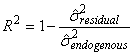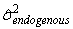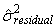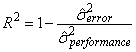﻿ How does Amos compute squared multiple correlations?

# How does Amos compute squared multiple correlations?

Amos computes a squared multiple correlation for each endogenous variable using the formula,

whereis the estimated (implied) variance of the endogenous variable andis the estimated (implied) variance of the associated residual variable.

For example, in the following path diagramthe squared multiple correlation for performance is estimated as.

It is not clear how to interpret squared multiple correlations in nonrecursive models. Alternative formulas for estimating the squared multiple correlation have been proposed. (See the references below.)

## References

Bentler, P., & Raykov, T. (2000). On measures of explained variance in nonrecursive structural equation models. Journal of Applied Psychology, 85(1), 125-131.

Jöreskog, J.G. (October 2, 1999). What is the interpretation of R2? (http://www.ssicentral.com/lisrel/techdocs/WhatistheinterpretationofR2.pdf)